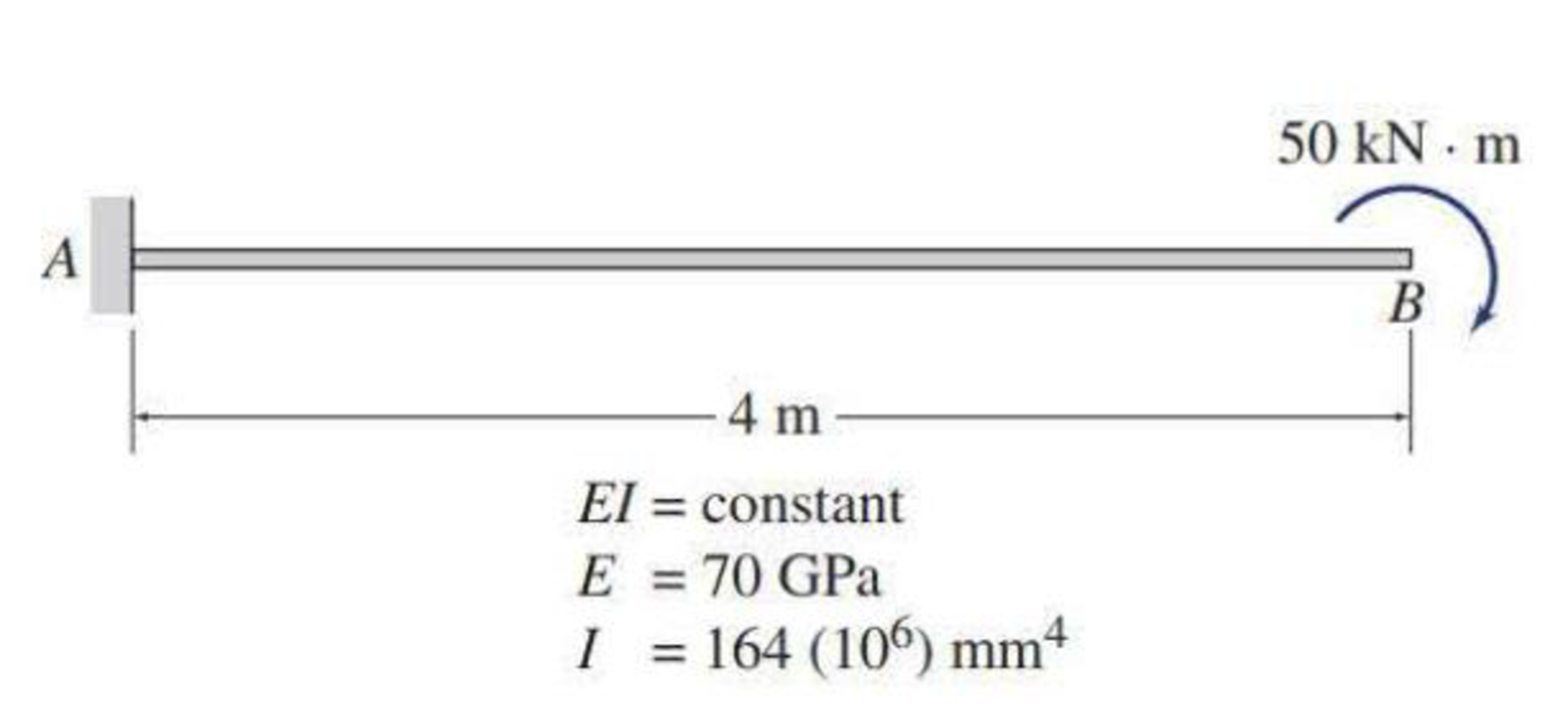# 7.57 and 7.58 Use Castigliano’s second theorem to determine the slope and deflection at point B of the beam shown in Figs. P7.20 and P7.21.

#### Solutions

Chapter
Section
Chapter 7, Problem 58P
Textbook Problem
11 views

## 7.57 and 7.58 Use Castigliano’s second theorem to determine the slope and deflection at point B of the beam shown in Figs. P7.20 and P7.21.To determine

Find the slope and deflection at point B of the beam using Castigliano’s second theorem.

### Explanation of Solution

Given information:

The beam is given in the Figure.

The value of E is 70 GPa and I is 164(106)mm4.

Apply the sign conventions for calculating reactions, forces and moments using the three equations of equilibrium as shown below.

• For summation of forces along x-direction is equal to zero (Fx=0), consider the forces acting towards right side as positive (+) and the forces acting towards left side as negative ().
• For summation of forces along y-direction is equal to zero (Fy=0), consider the upward force as positive (+) and the downward force as negative ().
• For summation of moment about a point is equal to zero (Matapoint=0), consider the clockwise moment as negative and the counter clockwise moment as positive.

Calculation:

Let apply a fictitious load P and couple M¯ at point B in the desired direction to find the deflection and slope.

The value of fictitious load P is zero.

Sketch the beam with fictitious load P and couple M¯ as shown in Figure 1.

Let the equation for bending moment at distance x in terms of load P and couple M¯ be M.

The couple M¯ is 50kNm.

Refer to Figure 1.

The equation for bending moment is,

M=M¯Px (1)

Substitute 0 for P and 50kNm for M¯.

M=50

Find the derivative MM¯ using Equation (1).

MM¯=1

The expression for slope at B using Castigliano’s second theorem (θB) is shown as follows:

θB=0L(MM¯)MEIdx

Here, L is the length of the beam.

Substitute 4 m for L, 1 for MM¯, and 50 for M.

θB=1EI04(1)(50)dx=1EI04(50)dx=1EI[50x]04=1EI(50×4)

θB=200EI

Substitute 70 GPa for E and 164(106)mm4 for I.

θB=200kNm2(70GPa)(164(106)mm4)=200kNm2(103N1kN)(70GPa×109N/m21GPa)(164(106)mm4×1016m41mm4)=0

### Still sussing out bartleby?

Check out a sample textbook solution.

See a sample solution

#### The Solution to Your Study Problems

Bartleby provides explanations to thousands of textbook problems written by our experts, many with advanced degrees!

Get Started

Find more solutions based on key concepts
For a building located in Baltimore, Maryland with annual heating degree-days (dd) of 4654, a heating load (hea...

Engineering Fundamentals: An Introduction to Engineering (MindTap Course List)

Identify the five phases in the SDLC. Name three guidelines for system development.

Enhanced Discovering Computers 2017 (Shelly Cashman Series) (MindTap Course List)

If such an e-mail came in, what would be the best action to take?

Principles of Information Security (MindTap Course List)

Create the relational diagram to show the relationship between DIRECTOR and PLAY.

Database Systems: Design, Implementation, & Management

After a weld is back gouged and a groove is formed, what should be done?

Welding: Principles and Applications (MindTap Course List)

If your motherboard supports ECC DDR3 memory, can you substitute non-ECC DDR3 memory?

A+ Guide to Hardware (Standalone Book) (MindTap Course List)